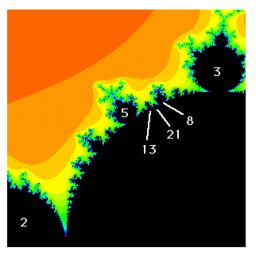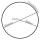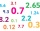# Sequence

Find the common ratio of the sequence -3, -1.5, -0.75, -0.375, -0.1875.

Ratio write as decimal number rounded to tenth.

Correct result:

q =  0.5

#### Solution:

$q=\frac{{a}_{n+1}}{{a}_{n}}=\frac{-1.5}{-3}=0.5$We would be pleased if you find an error in the word problem, spelling mistakes, or inaccuracies and send it to us. Thank you!Tips to related online calculators
Check out our ratio calculator.

## Next similar math problems:

• Decimal to fractionWrite decimal number 8.638333333 as a fraction A/B in the basic form. Given decimal has infinite repeating figures.
• Missing term 2What is the missing term for the Geometric Progression (GP) 3, 15, 75,__, 1875?
• Sequence - 5 membersWrite first five members of the sequence ?
• Circumference - a simpleWhat is the ratio of the circumference of any circle and its diameter? Write the result as a real number rounded to 2 decimal places.
• Series and sequencesFind a fraction equivalent to the recurring decimal? 0.435643564356
• Sum 1-6Find the sum of the geometric progression 3, 15, 75,… to six terms.
• Six termsFind the first six terms of the sequence a1 = -3, an = 2 * an-1
• Tenth memberCalculate the tenth member of geometric sequence when given: a1=1/2 and q=2
• Geometric sequence 5About members of geometric sequence we know: ? ? Calculate a1 (first member) and q (common ratio or q-coefficient)
• Five membersWrite first 5 members geometric sequence and determine whether it is increasing or decreasing: a1 = 3 q = -2
• Infinite decimalImagine the infinite decimal number 0.99999999 .. ... ... ... That is a decimal and her endless serie of nines. Determine how much this number is less than the number 1. Thank you in advance.
• Sequence 11What is the nth term of this sequence 1,1/2,1/3,1/4,1/5 ?
• Mystery of stereometrieTwo regular tetrahedrons have surfaces 88 cm2 and 198 cm2. In what ratio is their volumes? Write as a fraction and as a decimal rounded to 4 decimal places.
• What is one thirdWhat is 1/3 as a decimal? Give your answer rounded to 2 decimal places.
• Sequence 3Write the first 5 members of an arithmetic sequence: a4=-35, a11=-105.
• The accompanyingThe accompanying table gives the probability distribution of the number of courses randomly selected student has registered Number of courses 1 2 3 4 5 6 7 Probability 0.02 0.03 0.1 0.3 0.4 - 0.01 respectively. a) Find the probability of a student registe
• Geometric progression 2There is geometric sequence with a1=5.7 and quotient q=-2.5. Calculate a17.